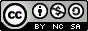Journal topic
Nonlin. Processes Geophys., 12, 643–660, 2005
https://doi.org/10.5194/npg-12-643-2005Special issue: Nonlinear plasma waves-solitons, periodic waves and...

Nonlin. Processes Geophys., 12, 643–660, 2005
https://doi.org/10.5194/npg-12-643-200522 Jun 2005

22 Jun 2005

# Hamiltonian formulation of nonlinear travelling Whistler waves

G. M. Webb1, J. F. McKenzie3,2,1, E. M. Dubinin4, and K. Sauer4 G. M. Webb et al.
• 1Institute of Geophysics and Planetary Physics, University of California Riverside, Riverside CA 92521, USA
• 2School of Physics and School of Mathematical and Statistical Sci., Univ. of KwaZulu-Natal, Durban, 4041, South Africa
• 3University of KwaZulu-Natal (Howard College), Durban, 4041, South Africa
• 4Max-Planck-Institute f¨ur Sonnensystemforschung, Katlenburg-Lindau, Germany

Abstract. A Hamiltonian formulation of nonlinear, parallel propagating, travelling whistler waves is developed. The complete system of equations reduces to two coupled differential equations for the transverse electron speedand a phase variablerepresenting the difference in the phases of the transverse complex velocities of the protons and the electrons. Two integrals of the equations are obtained. The Hamiltonian integral H, is used to classify the trajectories in thephase plane, whereand w=u2 are the canonical coordinates. Periodic, oscilliton solitary wave and compacton solutions are obtained, depending on the value of the Hamiltonian integral H and the Alfvén Mach number M of the travelling wave. The second integral of the equations of motion gives the position x in the travelling wave frame as an elliptic integral. The dependence of the spatial period, L, of the compacton and periodic solutions on the Hamiltonian integral H and the Alfvén Mach number M is given in terms of complete elliptic integrals of the first and second kind. A solitary wave solution, with an embedded rotational discontinuity is obtained in which the transverse Reynolds stresses of the electrons are balanced by equal and opposite transverse stresses due to the protons. The individual electron and proton phase variablesandare determined in terms ofand. An alternative Hamiltonian formulation in whichis the new independent variable replacing x is used to write the travelling wave solutions parametrically in terms of.